# Intermediate value theorem

## Statement

### Full version

Suppose$f$ is a continuous function and a closed interval$[a,b]$ is contained in the domain of$f$ (in particular, the restriction of$f$ to the interval$[a,b]$ is continuous). Then, for any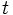$t$ between the values$f(a)$ and$f(b)$ (see note below), there exists$c \in [a,b]$ such that$f(c) = t$.

Note: When we say$t$ is between$f(a)$ and$f(b)$, we mean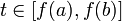$t \in [f(a),f(b)]$ if$f(a) \le f(b)$ and we mean that$t \in [f(b),f(a)]$ if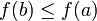$f(b) \le f(a)$.

### Short version

Any continuous function on an interval satisfies the intermediate value property.

## Caveats

### The statement need not be true for a discontinuous function

It is possible for a function having a discontinuity to violate the intermediate value theorem. Below is an example, of the function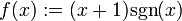$f(x) := (x + 1)\operatorname{sgn}(x)$ where$\operatorname{sgn}$ is the signum function and we define it to be zero at 0.

Here, we consider the domain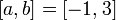$[a,b] = [-1,3]$, with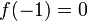$f(-1) = 0$ and$f(3) = 4$, but there is no$c \in [-1,3]$ satisfying$f(c) = 1/2$, even though$1/2 \in [0,4]$.

### The function needs to be defined throughout the domain

Consider the function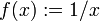$f(x) := 1/x$. We have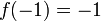$f(-1) = -1$ and$f(1) = 1$. However, there is no$c \in [-1,1]$ such that$f(c) = 1/2$. The reason the theorem fails is that$f$ is not defined at the point 0, and hence it is not defined on the domain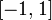$[-1,1]$.